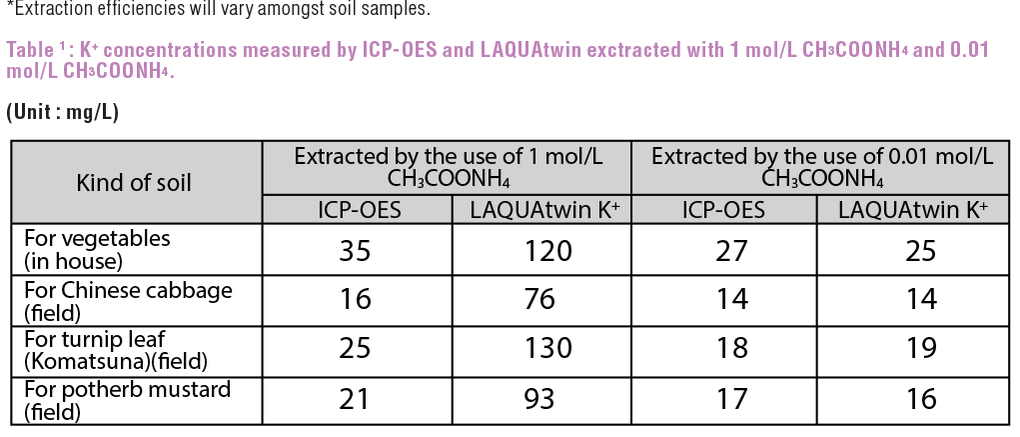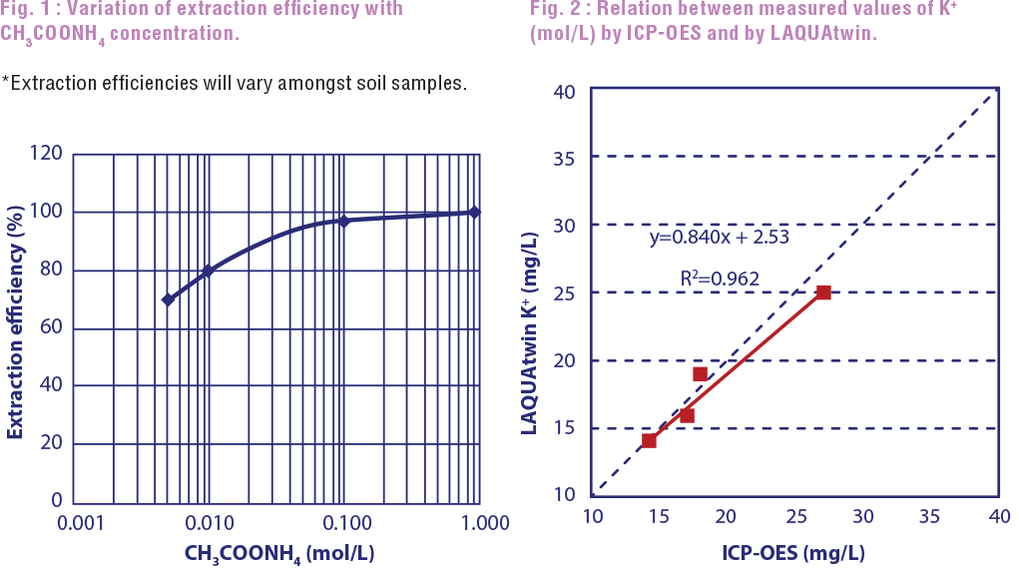# Measurement of Potassium in Soil

In laboratories, potassium in soil is extracted with 1M ammonium acetate and analyzed with Atomic Absorption (AA) or Inductivity Coupled Plasma-Optical Emission Spectrometry (ICP-OES). LAQUAtwin potassium ion meter showed values higher than those of ICP-OES. However, with 0.01M ammonium acetate extraction, good correlation (r=0.981, r2=0.962) was obtained between ICP-OES and LAQUAtwin potassium ion meter.#### Introduction

Typically, Atomic Absorption (AA) or Inductivity Coupled Plasma-Optical Emission Spectrometry (ICP-OES) is used to measure potassium ion, by first extracting the potassium ion from sample soils by 1 mol/L ammonium acetate (CH3COONH4). These are the methods performed in laboratories.

A simpler method for a rapid measurement of potassium ion in soil uses the LAQUAtwin potassium ion meter B-731. The extraction method is the same as the lab method. The following procedure explains how you can measure K+ with good correlation to analytical lab tests.

#### Method

1. Put 1g each of air-dried soils (four samples) in 100mL glass beakers, two beakers per soil sample.
2. Prepare two kinds of extraction per soil sample, one by adding 20 ml of 1mol/L CH3COONH4 to one beaker, and 20ml of 0.01mol/L CH3COONH4 to another beaker.
3. Shake the beakers around 1 hour to extract K+ from the soil using a bench top shaker.
4. Calibrate LAQUAtwin B-731 with 150mg/L and 2000mg/L K+ standard solutions included in the product.
5. Measure potassium ion concentration of the filtrated solution with calibrated B-731 and with ICP-OES (e.g. HORIBA Jobin Yvon. Model ULTIMA2).
6. Perform this measurement with 4 different samples.

#### Results and Benefits

The LAQUAtwin B-731 allows for a simple on site determination of potassium which provides accuracy close to laboratory techniques. Table 1 shows the results from ICP-OES and LAQUAtwin K+ meter extracted with 1 mol/L and 0.01 mol/L CH3COONH(Internal study by HORIBA labs, 2013).Based on Table 1, higher value against ICP-OES is detected by LAQUAtwin K+ with 1 mol/L CH3COONH4 extraction, due to strong interference by NH4+ of CH3COONH4. However, with 0.01 mol/L CH3COONH4 extraction, although the extraction efficiency is reduced by approximately 80%* (Figure 1), very good correlation (r=0.981, r2=0.962) is obtained between ICP-OES and LAQUAtwin (Figure 2).

Figure 1 shows the potassium extraction efficiency measured with ICP-OES. Setting 1 mol/L CH3COONH4 extraction as 100%, efficiency trend is plotted depending on different CH3COONH4 concentration. Figure 2 shows the correlation between ICP-OES and LAQUAtwin K+ measurements with 0.01 mol/L CH3COONH4 extraction.